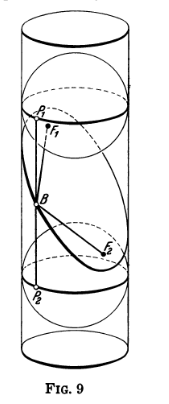The cross-section of a cylinder is an ellipse

Here’s an interactive visual diagram to accompany the proof of the elementary fact that the cross-section of a cylinder is an ellipse:

I was inspired to make this when I saw this illustration in Geometry and the Imagination.Given a cylinder with radius $r$, and the plane slicing through it, the proof goes as follows. Imagine the cylinder is hollow, with two spheres placed snugly (that is, of radius $r$) into it, so that they touch against the intersecting plane. The claim is that these points where the sphere touch the cross-section are the foci of the ellipse. We’ll show this is an ellipse by proving that the sum of the distance from the foci to any point on the boundary is constant.

Take an arbitrary point on the ellipse, $p$. Then let’s call the lengths of the two red lines $r_{v, p}$ and $r_{h, p}$ for the vertical red line and the “horizontal” (ellipse-bound) red line from $p$. The interactive visualization above loops over the values of $p$. Likewise, the lengths of the blue lines will be denoted $b_{v, p}$ and $b_{h, p}$.

Then notice that the two red lines in the visualization are both tangent to the sphere: the wall of the cylinder is tangent to the sphere at all points where they make contact, and the intersecting plane is tangent to the sphere.

Now recall (or convince yourself) that tangents from a sphere that intersect at the same point are of the same length. That is, $r_{v, p} = r_{h, p}$, and $b_{v, p} = b_{h, p}$ for all $p$.

Moreover, the two vertical lines always sum up to a constant: $r_{v, p} + b_{v, p} = K$ for all $p$.

Combining those two equations gives $r_{h, p} + b_{h, p} = K$ for all $p$, which shows that the cross-section is an ellipse.

I think it’s a testament to good mathematical illustration that the drawing from Geometry and the Imagination is easier to follow (for me) than the interactive 3d one. But there is something very neat about seeing these old diagrams “come to life”.

I made this using the library mathbox.